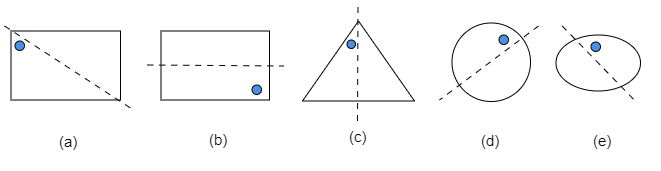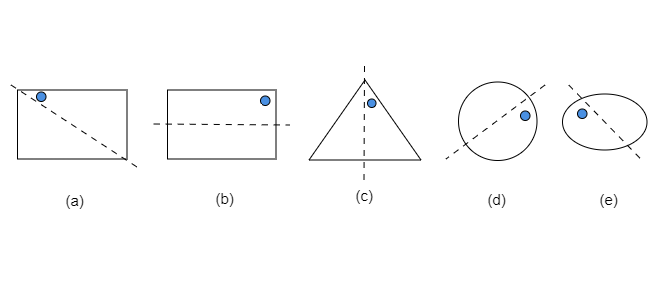"
">

# Given the line(s) of symmetry, find the other hole(s):"

Given: A line of symmetry and a hole is given in each shape in the given figure.

To do: We have to find the other hole. The other hole should be at that place such that if it is divided by any line of symmetry, it will divide it into equal parts.

Solution:

We will draw a line passing through the center point to each shape, in order to check their symmetry.Updated on: 10-Oct-2022

27 Views